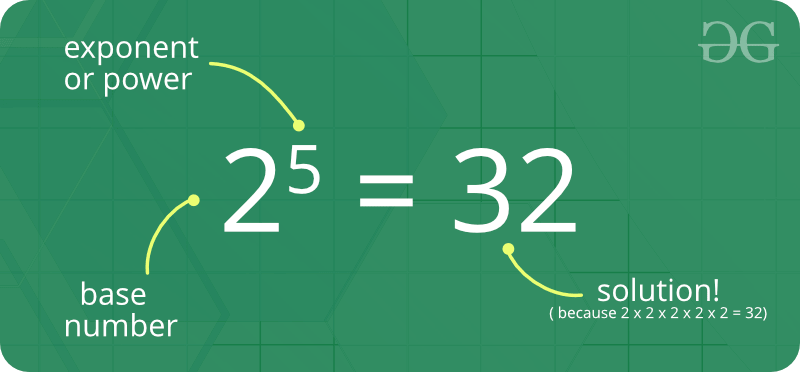# Power in Mathematics

The power of a number says how many times to use the number in a multiplication. Powers are also called Exponents or Indices. For example, 8^2 could be called “8 to the power 2” or “8 to the second power”, or simply “8 squared”.Some interesting fact about Power :

1. If the indices is 1, then you just have the number itself. For example, 5^1 = 5
2. If the indices is 0, then you get 1. For example, 5^0 = 1
3. Exponents make it easier to write and use many multiplications
4. Negative exponent means how many times to divide one by the number.For example, 5^-1 = 1 /5 = 0.2

How we check if a number is power of y for a given integer x ?

Naive solution:
Given two positive numbers x and y, check if y is a power of x or not.

Examples :

```Input:  x = 10, y = 1
Output: True

Input:  x = 10, y = 1000
Output: True

Input:  x = 10, y = 1001
Output: False
```

Approach : A simple solution is to repeatedly compute powers of x. If a power becomes equal to y, then y is a power, else not.

## C++

 `// C++ program to check if a number is power of ` `// another number ` `#include ` `using` `namespace` `std; ` ` `  `/* Returns 1 if y is a power of x */` `bool` `isPower(``int` `x, ``long` `int` `y) ` `{ ` `    ``// The only power of 1 is 1 itself ` `    ``if` `(x == 1) ` `        ``return` `(y == 1); ` ` `  `    ``// Repeatedly comput power of x ` `    ``long` `int` `pow` `= 1; ` `    ``while` `(``pow` `< y) ` `        ``pow` `*= x; ` ` `  `    ``// Check if power of x becomes y ` `    ``return` `(``pow` `== y); ` `} ` ` `  `/* Driver program to test above function */` `int` `main() ` `{ ` `    ``cout << (isPower(10, 1) ? ``"True"` `: ``"False"``) << endl; ` `    ``cout << (isPower(1, 20) ? ``"True"` `: ``"False"``) << endl; ` `    ``cout << (isPower(2, 128) ? ``"True"` `: ``"False"``) << endl; ` `    ``cout << (isPower(2, 30) ? ``"True"` `: ``"False"``) << endl; ` `    ``return` `0; ` `} `

## Java

 `// Java program to check if a number is power of ` `// another number ` `public` `class` `Test { ` ` `  `    ``// driver method to test power method ` `    ``public` `static` `void` `main(String[] args) ` `    ``{ ` `        ``// check the result for true/false and print. ` `        ``System.out.println(isPower(``10``, ``1``) ? ``"True"` `: ``"False"``); ` `        ``System.out.println(isPower(``1``, ``20``) ? ``"True"` `: ``"False"``); ` `        ``System.out.println(isPower(``2``, ``128``) ? ``"True"` `: ``"False"``); ` `        ``System.out.println(isPower(``2``, ``30``) ? ``"True"` `: ``"False"``); ` `    ``} ` ` `  `    ``/* Returns true if y is a power of x */` `    ``public` `static` `boolean` `isPower(``int` `x, ``int` `y) ` `    ``{ ` `        ``// The only power of 1 is 1 itself ` `        ``if` `(x == ``1``) ` `            ``return` `(y == ``1``); ` ` `  `        ``// Repeatedly compute power of x ` `        ``int` `pow = ``1``; ` `        ``while` `(pow < y) ` `            ``pow = pow * x; ` ` `  `        ``// Check if power of x becomes y ` `        ``return` `(pow == y); ` `    ``} ` `} `

## Python3

 `# Python program to check ` `# if a number is power of ` `# another number ` ` `  `# Returns true if y is a ` `# power of x  ` `def` `isPower (x, y): ` `     `  `    ``# The only power of 1 ` `    ``# is 1 itself ` `    ``if` `(x ``=``=` `1``): ` `        ``return` `(y ``=``=` `1``) ` `         `  `    ``# Repeatedly compute ` `    ``# power of x ` `    ``pow` `=` `1` `    ``while` `(``pow` `< y): ` `        ``pow` `=` `pow` `*` `x ` ` `  `    ``# Check if power of x ` `    ``# becomes y ` `    ``return` `(``pow` `=``=` `y) ` `     `  `     `  `# Driver Code ` `# check the result for ` `# true/false and print. ` `if``(isPower(``10``, ``1``)): ``print``(``"True"``) ` `else``: ``print``(``"False"``) ` ` `  `if``(isPower(``1``, ``20``)): ``print``(``"True"``) ` `else``: ``print``(``"False"``) ` ` `  `if``(isPower(``2``, ``128``)): ``print``(``"True"``) ` `else``: ``print``(``"False"``) ` ` `  `if``(isPower(``2``, ``30``)): ``print``(``"True"``) ` `else``: ``print``(``"False"``) ` `    `

## C#

 `// C# program to check if a number  ` `// is power of another number ` `using` `System; ` ` `  `class` `GFG ` `{ ` `     `  `    ``// Returns true if y is a power of x  ` `    ``public` `static` `bool` `isPower (``int` `x, ``int` `y) ` `    ``{ ` `        ``// The only power of 1 is 1 itself ` `        ``if` `(x == 1) ` `        ``return` `(y == 1); ` ` `  `        ``// Repeatedly compute power of x ` `        ``int` `pow = 1; ` `        ``while` `(pow < y) ` `        ``pow = pow * x; ` ` `  `        ``// Check if power of x becomes y ` `        ``return` `(pow == y); ` `    ``} ` `     `  `    ``// Driver Code ` `    ``public` `static` `void` `Main () ` `    ``{ ` `        ``//check the result for true/false and print. ` `        ``Console.WriteLine(isPower(10, 1) ? ``"True"` `: ``"False"``); ` `        ``Console.WriteLine(isPower(1, 20) ? ``"True"` `: ``"False"``); ` `        ``Console.WriteLine(isPower(2, 128) ? ``"True"` `: ``"False"``); ` `        ``Console.WriteLine(isPower(2, 30) ? ``"True"` `: ``"False"``); ` `    ``} ` `     `  `} `

## PHP

 ` `

Output:

```True
False
True
False
```

Time complexity of above solution is O(Logxy)

Basic Program related to Power :

More problems related to Powers :

Attention reader! Don’t stop learning now. Get hold of all the important DSA concepts with the DSA Self Paced Course at a student-friendly price and become industry ready.

My Personal Notes arrow_drop_upIf you like GeeksforGeeks and would like to contribute, you can also write an article using contribute.geeksforgeeks.org or mail your article to contribute@geeksforgeeks.org. See your article appearing on the GeeksforGeeks main page and help other Geeks.

Please Improve this article if you find anything incorrect by clicking on the "Improve Article" button below.